# Multiple Density Plots with Pandas in Python

• Last Updated : 03 Jan, 2021

Multiple density plots are a great way of comparing the distribution of multiple groups in your data.  We can make multiple density plots using pandas plot.density() function. However, we need to convert data in a wide format if we are using the density function. Wide data represents different groups in different columns. We convert data in a wide format using Pandas pivot() function.

Let’s create the simple data-frame and then reshape it into a wide-format:

Example 1:

Here we are using this data set.

Step 1: Creating dataframe from data set.

## Python3

 `import` `pandas as pd ` ` `  `# creating a dataframe ` `df ``=` `pd.read_csv(r``"gapminder1.csv"``) ` `df.head()`

Output: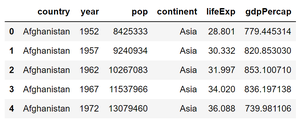dataset

Step 2: Let’s group data according to countries in different columns so that we can apply the density() function to plot multiple density plots.

## Python3

 `# converting data into wide-format ` `data_wide ``=` `df.pivot(columns``=``'continent'``, ` `                     ``values``=``'lifeExp'``) ` `data_wide.head() `

Output: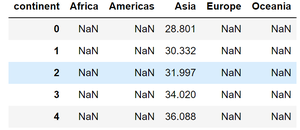Step 3: Now let’s plot multiple density plot using plot.density()

## Python3

 `import` `matplotlib.pyplot as plt ` ` `  `# calling density() to make ` `# multiple density plot  ` `data_wide.plot.density(figsize ``=` `(``7``, ``7``), ` `                       ``linewidth ``=` `4``) ` ` `  `plt.xlabel(``"life_Exp"``)`

Output :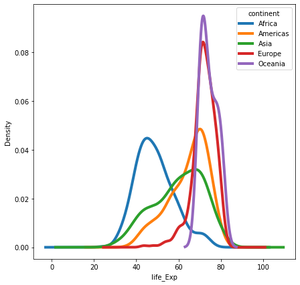Multiple density plots

Example 2: We can also call plot.kde() function on dataframe to make multiple density plots with Pandas.

Here we are using the tips dataset for this example, You can find it here.

Step 1: Creating dataframe from data set.

## Python3

 `import` `pandas as pd ` ` `  `# creating a dataframe ` `df ``=` `pd.read_csv(r``"tips.csv"``) ` `df.head() `

Output: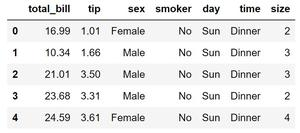tips_df

Step 2: Now apply pivot() function to have dataframe in the wide-format then apply kde() to have multiple density plot.

## Python3

 `# Converting to wide dataframe ` `data_wide ``=` `df.pivot(columns ``=` `'day'``, ` `                     ``values ``=` `'total_bill'``) ` ` `  `# plotting multiple density plot ` `data_wide.plot.kde(figsize ``=` `(``8``, ``6``), ` `                   ``linewidth ``=` `4``)`

Output: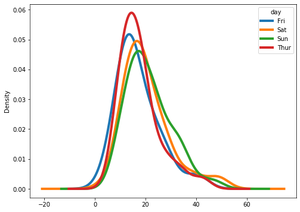tips multiple D.P

My Personal Notes arrow_drop_up
Recommended Articles
Page :